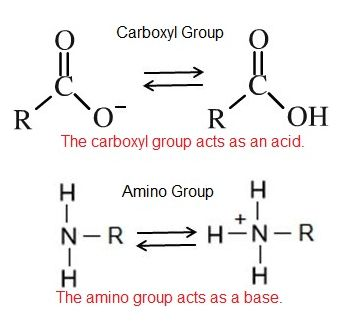Skip to main content

# pH#

## What is the role of Acid/Base Chemistry in Bis2A?We have learned that the behavior of chemical functional groups depends greatly on the composition, order and properties of their constituent atoms. As we will see, some of the properties of key biological functional groups can be altered depending on the pH (hydrogen ion concentration) of the solution that they are bathed in.

For example, some of the functional groups on the amino acid molecules that make up proteins can exist in different chemical states depending on the pH. We will learn that the chemical state of these functional groups in the context of a protein can have have a profound effect on the shape of protein or its ability to carry out chemical reactions. As we move through the course we will see numerous examples of this type of chemistry in different contexts.

pH is formally defined as:

$pH = -\log_{10} [H^+]$

In the equation above, the square brackets surrounding $$H^+$$ indicate concentration. If necessary, try a math review at wiki logarithm or kahn logarithm. Also see: concentration dictionary or wiki concentration.

Hydrogen ions are spontaneously generated in pure water by the dissociation (ionization) of a small percentage of water molecules into equal numbers of hydrogen (H+) ions and hydroxide (OH-) ions. While the hydroxide ions are kept in solution by their hydrogen bonding with other water molecules, the hydrogen ions, consisting of naked protons, are immediately attracted to un-ionized water molecules, forming hydronium ions (H30+).

Still, by convention, scientists refer to hydrogen ions and their concentration as if they were free in this state in liquid water. This is another example of a shortcut that we often take - it's easier to write H+ rather than H3O+. We just need to realize that this shortcut is being taken; otherwise confusion will ensue.Figure 1: Water spontaneously dissociates into a proton and hydroxyl group. The proton will combine with a water molecule forming a hydronium ion.
Attribution: Marc T. Facciotti

The pH of a solution is a measure of the concentration of hydrogen ions in a solution (or the number of hydronium ions). The number of hydrogen ions is a direct measure of how acidic or how basic a solution is.

The pH scale is logarithmic and ranges from 0 to 14 (Figure 2). We define pH=7.0 as neutral. Anything with a pH below 7.0 is termed acidic and any reported pH above 7.0 is termed alkaline or basic. Extremes in pH in either direction from 7.0 are usually considered inhospitable to life, although examples exist to the contrary. pH levels in the human body usually range between 6.8 and 7.4, except in the stomach where the pH is more acidic, typically between 1 and 2.Figure 2: The pH scale ranging from acidic to basic with various biological compounds or substances that exist at that particular pH. Attribution: Marc T. Facciotti

For additional information:

Watch this video for an alternative explanation of pH and its logarithmic scale.

The concentration of hydrogen ions dissociating from pure water is 1 × 10-7 moles H+ ions per liter of water.

1 mole (mol) of a substance (which can be atoms, molecules, ions, etc), is defined as being equal to 6.02 x 1023 particles of the substance. Therefore, 1 mole of water is equal to 6.02 x 1023 water molecules. The pH is calculated as the negative of the base 10 logarithm of this unit of concentration. The log10 of 1 × 10-7 is -7.0, and the negative of this number yields a pH of 7.0, which is also known as neutral pH.

Non-neutral pH readings result from dissolving acids or bases in water. High concentrations of hydrogen ions yields a low pH number, whereas low levels of hydrogen ions result in a high pH.

This inverse relationship between pH and the concentration of protons confuses many students - take the time to convince yourself that you "get it."

An acid is a substance that increases the concentration of hydrogen ions (H+) in a solution, usually by having one of its hydrogen atoms dissociate. For example, we have learned that the carboxyl functional group is an acid. The hydrogen atom can dissociate from the oxygen atom resulting in a free proton and a negatively charged functional group. A base provides either hydroxide ions (OH) or other negatively charged ions that combine with hydrogen ions, effectively reducing the H+ concentration in the solution and thereby raising the pH. In cases where the base releases hydroxide ions, these ions bind to free hydrogen ions, generating new water molecules. For example, we have learned that the amine functional group is a base. The nitrogen atom will accept hydrogen ions in solution, thereby reducing the number of hydrogen ions which raises the pH of the solution.Figure 3: The carboxylic acid group acts as an acid by releasing a proton into solution. This increases the number of protons in solution and thus decreases the pH. The amino group acts as a base by accepting hydrogen ions from solution, decreasing the number of hydrogen ions in solutions, thus increasing the pH.
Attribution: Erin Easlon

### Additional pH resources

Here are some additional links on pH and pKa to help learn the material. Note that there is an additional module devoted to pKa.